# Optimal design of protective clothing based on difference equation

## Pan Hu1, Heng Tang2, Ling Zheng3, Ci Jun Fang4

1, 2, 3Hubei University of Technology, Wuhan, P. R. China

4School of Science, Hubei University of Technology, Wuhan, P. R. China

4Corresponding author

Mathematical Models in Engineering, Vol. 5, Issue 2, 2019, p. 48-55. https://doi.org/10.21595/mme.2019.20752
Received 25 April 2019; received in revised form 16 May 2019; accepted 29 May 2019; published 30 June 2019

Copyright © 2019 Pan Hu, et al. This is an open access article distributed under the Creative Commons Attribution License, which permits unrestricted use, distribution, and reproduction in any medium, provided the original work is properly cited.
Editor's Pick
Views 168
CrossRef Citations 0
Abstract.

The temperature distribution and thickness design of high temperature protective clothing are studied in this paper. Based on the data provided by China mathematical modeling competition in 2018. We establish the temperature distribution model and skin layer heat conduction and burn model. The interface continuous conditional difference method, differential iterative method, least squares method and the chasing method are used to solve the given temperature distribution on the protective clothing in the environment, and analyze protective clothing meeting the actual needs.

Keywords: heat transfer equation, heat exchange coefficient, least squares, the chasing method.

#### 1. Introduction

When working in a high temperature environment, workers often need to wear adiabatic protective clothing, which can effectively prevent heatstroke, burns and other injuries, often have the characteristics of flame retardancy, liquid repellency, etc. Many researchers have carried out the material and thickness of the garment. Designed to increase the thermal insulation performance.

Lu studied the performance of protective clothing against liquid splash and its permeability and heat and moisture conductivity in high temperature environment, and used skin burn prediction model to predict the protection of thermal protective clothing . Tian et al. applied numerical simulation to evaluate the performance of high temperature protective clothing, and summarized the characteristics and disadvantages of typical heat conduction model and skin burn model .

Zhang et al analyzed the development status and defects of temperature-adjustable protective clothing at home and abroad, and analyzed the mechanism of phase change materials in protective clothing, and explained its application in protective clothing .

For the application of phase change materials, Zhu and others conducted more in-depth

research, analyzing from the aspects of reducing thermal stress, improving the comfort of protective clothing, preventing sudden changes in temperature, etc. . Zhang Chao et al took into account human physiological indicators, used the dummy model to simulate the heated scene, and established a human-clothing-environmental thermal protective clothing evaluation standard . Lin Jianbo studied the radiant heat penetration resistance of thermal protective clothing and selected two suitable thermal protective clothing materials . M. J. Slapak contrasts PBI fibers with other insulating materials to highlight their superiority as a thermal protective clothing material .

Yang analyzed the research status of thermal protective clothing in the police field and introduced the development status of the new thermal protective material aerogel . Li used 13 kinds of flame retardant fabrics as experimental samples, using statistical methods to obtain protective performance and fabric thickness, areal density, $tpp$ value is positive related . Shen Lanpin designed a double-layer thermal protective clothing fabric from the end use of flame retardant thermal protective clothing . Wang Weiwei et al used the improved finite difference method to establish a heat transfer model and optimized the thickness of each layer of thermal protective clothing . Lu Linzhen calculated the temperature value of each fixed contact surface with time, predicted the critical time of the burn level, and prevented skin burns .

In this paper, the temperature distribution outside the protective clothing is studied with time and distance. Lu Linzhen also carried out related research and established the heat transfer model of the protective clothing-air layer-skin system, and the influence of some parameters on the protective performance . In addition, this paper optimizes the thickness of the garment in a specific environment to achieve the best protection.

#### 2. Protective clothing temperature distribution model

The protective clothing studied in this paper is divided into four layers. A straight line of vertical protective clothing is selected as the research object. The outer side of the first layer is taken as the origin, and the horizontal direction is directed to the positive direction of the skin layer to establish the $x$-axis. The arrangement relationship of each layer is shown in Fig. 1. As shown, it is assumed that the layers are tightly connected and the density of the medium is uniform, wherein the thickness of the $m$th layer is recorded as ${l}_{m}$ (1, 2, 3, 4), and ${L}_{m}=\sum _{k=1}^{m}{l}_{k}$.

Fig. 1. The arrangement relationship of each layer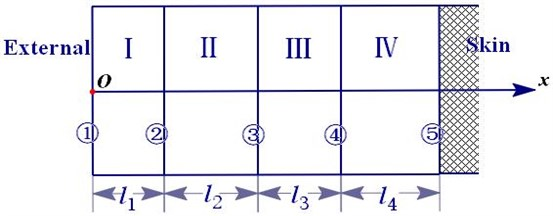#### 2.1. Generalized equation

(1)

the temperature distribution function of each layer is ${T}_{m}\left(x,t\right)$ ($m=$ 1, 2, 3, 4) where ${a}_{m}^{2}={k}_{m}/{c}_{m}{\rho }_{m}$, ${c}_{m}$ is the specific heat capacity of the $m$th layer, ${\rho }_{m}$ is the density of the $m$th layer, ${k}_{m}$ is the thermal conductivity of the $m$th layer. The analysis of the conditions for the solution of the above generalized equations is as following.

#### 2.2. Initial conditions

Assume that the initial temperature of each point of the protective suit is the same, so there are initial conditions:

(2)
$T\left(x,0\right)={w}_{0},$

where ${w}_{0}$ is the temperature of the protective clothing before entering the laboratory, the protective clothing has been worn before entering the laboratory, so it is assumed that the temperature is equal to the human body temperature.

#### 2.3. Boundary conditions

For the actual situation of the protective layer and the contact surface, we use the third type of boundary conditions and interface continuous conditions.

According to the Heat Transfer theory, when there is heat exchange between the solid and the fluid, the third type of boundary condition is used:

(3)

where $T\left(l,\mathrm{\infty }\right)$ is a stable temperature maintained by the left boundary of the first layer after a long period of time, $h$ is the heat exchange coefficient that is a constantdetermined by the material on both sides of the contact surface.

When the two objects are in full contact, the temperature of the contact surfaces of the two objects and the heat flux density values are equal:

$-{k}_{1}\frac{\partial {T}_{1}}{\partial x}\left|\begin{array}{l}\\ x=l\end{array}=-{k}_{2}\right\frac{\partial {T}_{2}}{\partial x}\left|\begin{array}{l}\\ x=l\end{array}\right.$

#### 2.4. The left end boundary condition of the first layer

For the left boundary of the $i$th layer (1 in Fig. 1), the left side is air fluid, and the right side is the $i$th layer of clothing material, then:

$-{k}_{1}\frac{\partial {T}_{1}}{\partial x}\left|\begin{array}{l}\\ x=0\end{array}={h}_{1}\left({w}_{1}-T\left(0,t\right)\right)\right,$

among them ${h}_{1}$ is the heat exchange coefficient between the $i$th layer and the air, ${w}_{1}$ is the outside temperature, ${w}_{1}=\text{75}$.

#### 2.5. Contact surface boundary condition

For $x={L}_{m}$, two sides are solid clothing materials, there are temperature equations:

(4)

Heat flux density equation:

(5)

#### 2.6. The IV layer right end boundary condition

For the right edge of the iv layer (5 in Fig. 1), there is a third type of boundary condition:

(6)
$-{k}_{4}\frac{\partial {T}_{4}}{\partial x}\left|\begin{array}{l}\\ x={L}_{4}\end{array}={h}_{2}\left({w}_{2}-T\left({L}_{4},t\right)\right)\right,$

where ${w}_{2}$ is body temperature.

A partial differential equation model of the temperature distribution of the protective suit is obtained from the above Eqs. (1)-(5).

#### 3.1. Difference method

To solve the differential equation of Eq. (1), convert the differential equation into a difference equation. The table represents the coordinate $x$ on the horizontal axis and the time $t$ on the vertical axis. The content is the temperature at the corresponding coordinate $x$ and time $t$, temperature function $T\left(x,t\right)$. ${T}_{i,j}$ indicates the temperature corresponding to the $j$ coordinate $x$ at the $i$ time $t$, as shown in Fig. 2.

Fig. 2. Time-coordinate table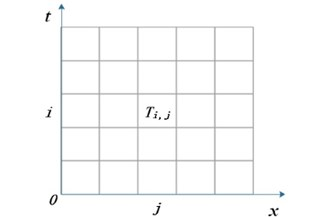#### 3.1.1. Heat conduction equation difference

For the solution of differential equations, it is often considered to differentiate the differential equations. The partial derivatives of the differential equations of Eq. (1) can be transformed.

From the first-order backward difference quotient and the second-order center difference:

${c}_{m}{\rho }_{m}\cdot \frac{{T}_{i,j}-{T}_{i-1,j}}{\mathrm{\Delta }t}={k}_{m}\frac{{T}_{i,j+1}-2{T}_{i,j}+{T}_{i,j-1}}{\mathrm{\Delta }{x}_{m}^{2}}$

among them ${\lambda }_{m}={k}_{m}\mathrm{\Delta }t/{c}_{m}{\rho }_{m}\mathrm{\Delta }{x}_{m}^{2}$.

#### 3.1.2. The third type of boundary condition difference method

For the third type of boundary conditions, the difference is used to discretize the boundary conditions, and the boundary temperature is calculated.

At the left and right edge:

(7)
$\left\{\begin{array}{l}-{k}_{1}\frac{\partial u}{\partial x}+{h}_{1}T={h}_{1}{w}_{1},\\ {k}_{4}\frac{\partial u}{\partial x}+{h}_{2}T={h}_{2}{w}_{2},\end{array}\right\$
$\left\{\begin{array}{l}\left(1+{M}_{1}\right){T}_{i,0}-{T}_{i,1}={M}_{1}{w}_{1},\\ -{T}_{i,n-1}+\left(1+{M}_{2}\right){T}_{i,n}={M}_{2}{w}_{2},\end{array}\right\$

among them ${M}_{1}={h}_{1}/{k}_{1}\cdot \mathrm{\Delta }{x}_{1}$, ${M}_{2}={h}_{2}/{k}_{4}\cdot \mathrm{\Delta }{x}_{4}$, $n$ is the total number of columns.

#### 3.1.3. Interface continuous condition difference

At the interface of different materials, because the parameters on both sides are different, and the heat conduction equation is different, the temperature value cannot be obtained by Eq. (3). In this paper, the difference in cross-section temperature and the equal heat flux density are considered, and the difference is symmetrized to obtain the interface. It is temperature function differentiation method:

(8)
${k}_{m}\frac{{T}_{i,j}-{T}_{i,j-1}}{\mathrm{\Delta }{x}_{m}}={k}_{m+1}\frac{{T}_{i,j+1}-{T}_{i,j}}{\mathrm{\Delta }{x}_{m+1}},$
$-{N}_{m}{T}_{i,j-1}+\left({N}_{m}+{N}_{m+1}\right){T}_{i,j}-{N}_{m+1}{T}_{i,j+1}=0,$

among them ${N}_{m}={k}_{m}/\mathrm{\Delta }{x}_{m}$.

Connect the Eqs. (6), (7) and (8) in parallel, transform into a linear system of equations, and use the chasing method to solve the equations.

#### 3.2. Solution of heat exchange coefficient

The difference iterative method in 2.1 was used to obtain ${T}_{i,n}$, combining with the measured values ${T}^{\mathrm{*}}$in annex 2, and the least square method was used to solve the following optimization problems:

Finally, use the chasing method to obtain parameters that 135.094 m2/s and 8.366 m2/s, respectively.

The known parameters used (all have been converted to standard units): ${w}_{1}=$ 75, ${w}_{2}=$ 37, ${l}_{1}=$ 6×10-4, ${l}_{2}=$ 6×10-3, ${l}_{3}=$ 3.6×10-3, ${l}_{4}=$ 5×10-3.

#### 3.3. Temperature distribution

Use the obtained parameters ${h}_{1}$, ${h}_{2}$, and the differential iteration method in 2.1, we find the temperature distribution of each point at each moment as shown in Fig. 3, Fig. 4 and the boundary temperature of each layer at each moment in Fig. 5.

Fig. 3. Two-dimensional graph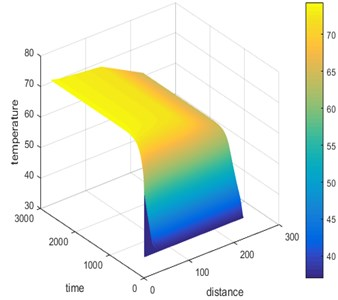Fig. 4. Three-dimensional graph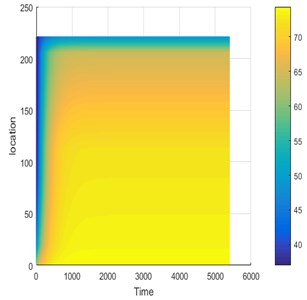According to Fig. 3 and Fig. 4, it can be found that the temperature of the protective clothing changes monotonously with the distance and time from the origin, and finally stabilizes, that is, the ambient temperature given by the problem is 75 °C, which basically conforms to the actual situation.

Fig. 5. The boundary temperature of each layer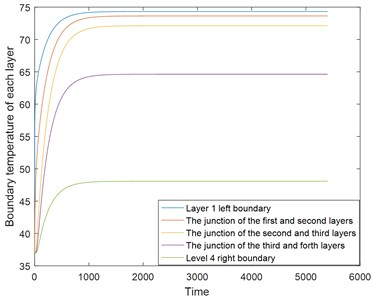#### 4. Skin layer heat conduction and burn model

In order to further determine the optimal thickness of the thermal protective suit, it is first necessary to determine the temperature value of the skin layer, so it is considered to add a layer on the right side of the skin surface to be the skin layer. We analyze generalized equation, boundary conditions and initial conditions of the skin layer as follows.

#### 4.1. Generalized equation

The Pennes biothermal transfer model introduced in the literature  was determined as:

$\frac{\partial {T}_{5}}{\partial t}=\frac{{k}_{5}}{{c}_{5}{\rho }_{5}}\frac{{\partial }^{2}{T}_{5}}{\partial {x}^{2}}-\frac{{c}_{b}{\rho }_{b}{w}_{b}}{{c}_{5}{\rho }_{5}}\left({T}_{r}-{T}_{5}\right),$

among them ${k}_{5}$ is the thermal conductivity of the skin, ${w}_{b}$ is infusing blood, ${c}_{5}$, ${\rho }_{5}$ are the density and specific heat of the skin layer, ${c}_{b}{\rho }_{b}{w}_{b}\left({T}_{r}-{T}_{5}\right)$ represents the energy of heat exchange between human cells and blood.

#### 4.2. Boundary conditions

The left side of the skin layer is the interface between the air layer and the skin surface, and may involve thermal radiation, and the heat radiation gradually decays from the first layer toward the skin layer:

where $\eta$ is Attenuation coefficient.

The right border is a constant temperature of 37 ℃ in the human body, 37.

#### 4.3. Initial conditions

At the initial moment, the temperature is 37 ℃ everywhere, .

Establish the heat transfer model of the 5th layer:

The above equation is differentiated by the forward difference method, the skin surface temperature is calculated from the difference expression and the initial condition and the boundary condition, and the squared sum of difference of the theoretical value and the measured value is used to determine the parameter in the skin layer heat transfer model.

In order to make the research meet the actual needs, we analyze the degree of burns :

$\mathrm{\Omega }\left(x,\tau \right)=\mathrm{\Omega }\left(x,0\right)+{\int }_{0}^{\tau }P{e}^{-\frac{\mathrm{\Delta }E}{R\left(T\left(x,t\right)+273\right)}}dt,$

among them $\mathrm{\Delta }E$ is skin activation properties, $P$ is the frequency disruption factor.

When $\mathrm{\Omega }\le \text{0.53,}$ no burns will occur. $\text{0.53}<\mathrm{\Omega }\le \text{1,}$ a first-degree burn occurs, $\text{0.53}<\mathrm{\Omega }\le$ 104, second-degree burn occurs. $\mathrm{\Omega }>$ 104, third-degree burn occurs.

#### 5. Sensitivity analysis

Since the data given by the accessory is measured, the measurement error generally shows a normal distribution. Sensitivity analysis is now performed on the model. Add the raw data to the measurement error with a mean of 0.01 and a variance of 0.01 and recalculate. When $t=\text{2000}$, a temperature along with time $t$ changing image is drawn. When $x=\text{200}$, a temperature along with distance $x$ changing image is drawn.

Fig. 6. Sensitivity analysis chart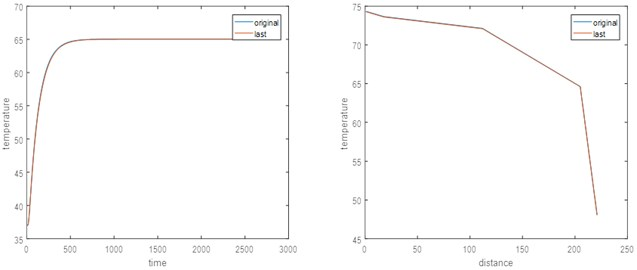It is known from the image that when considering the measurement error, the calculated temperature value is not much different from the temperature value calculated from the original measurement data, and only some random fluctuations are generated on the basis of the original data, and the overall trend of the image does not change much. Therefore, the model and algorithm we have established are highly stable.

#### 6. Conclusions

In this paper, a temperature distribution model is established for thermal protective clothing at high temperature. The temperature distribution of each layer of thermal protection at a specific ambient temperature is studied, and the optimal thickness of each layer is obtained by combining specific temperature and thickness constraints. In the real environment, it is necessary to consider factors such as changes in the influence of human sweat and the moisture contained in the fabric itself, and it is possible to establish a heat and moisture transfer model under transient conditions in a multi-layer fabric combination state, and The radiant heat transfer between the layers is studied in depth, and the influence of the initial water content is analyzed, and the changes in the physical parameters affected by the water content in the model are analyzed.

1. Lu Yehu Prediction of Heat and Moisture Transfer and Skin Burn in Thermal Protective Clothing under High Temperature Liquid Environment. Donghua University, 2013. [Search CrossRef]
2. Tian Miao, Li Jun Application of numerical simulation in performance evaluation of thermal protective clothing. Journal of Textiles, Vol. 36, Issue 1, 2015, p. 158-164. [Search CrossRef]
3. Zhang Dongxia, Guo Fengzhi Application of phase change materials in temperature control clothing. Knitting Industry, Vol. 3, 2007, p. 28-31. [Search CrossRef]
4. Zhu Fanglong, Fan Jianbin, Feng Qianqian, Zhou Yu Application and feasibility analysis of phase change materials in fire fighting suits. Journal of Textile Research, Vol. 35, Issue 8, 2014, p. 124-132. [Search CrossRef]
5. Zhang Chao, Qin Tingxin, Wang Jinyu Discussion on evaluation standards of overall protective effect of thermal protective clothing based on human physiological indexes. Standard Science, Vol. 9, 2013, p. 43-46. [Search CrossRef]
6. Lin Jianbo, Yin Haibo, Cao Yongqiang Radiation resistance of firefighters' thermal protective clothing. Fire Science and Technology, Vol. 34, Issue 2, 2015, p. 241-243. [Search CrossRef]
7. Slapak M. J., Yan Cheng PBI fiber used for making heat-resistant protective clothing. Foreign Textile Technology (Chemical Fiber, Dyeing and Finishing, Environmental Protection Volume), Vol. 3, 1992, p. 6-11. [Search CrossRef]
8. Yang Jie, Qiu Rixiang Application research and development of police thermal protection materials. China Personal Protective Equipment, Vol. 4, 2012, p. 5-9. [Search CrossRef]
9. Li Hongyan, Wu Xuanrun, Zhang Yuyuan, Yang Kai Relationship between fabric properties and comprehensive protection ability of thermal protective clothing. Journal of Textiles, Vol. 9, Issue 59, 2008, p. 61-71. [Search CrossRef]
10. Shen Lanping, Li Yiling, Pan Hairong, Fan Lihong Development of double-layer flame-retardant and heat-resistant protective clothing fabrics. Beijing Textile, Vol. 1, 2000, p. 46-47. [Search CrossRef]
11. Wang Weiwei, Wang Cheng, Pan Yuyue, Wang Hui Optimization design of high temperature protective clothing based on Fourier's law of heat conduction. Electronic Test, Vol. 23, 2018, p. 53-55. [Search CrossRef]
12. Lu Linzhen, Xu Dinghua, Xu Yinhong Prediction of skin burn degree using an improved heat transfer model of three-layer thermal protective clothing. Textile Journals, Vol. 39, Issue 1, 2008, p. 111-118+125. [Search CrossRef]
13. Lu Linzhen Heat Transfer Model and Optimal Parameters of Multi-Layer Thermal Protective Clothing. Zhejiang University of Science and Technology, 2018. [Search CrossRef]
14. Chen Qinghua, Dong Changshuai, Ma Yan, Pang Li, Liu Zegong Measurement of thermal properties of solid materials based on one-dimensional unsteady heat transfer model with constant temperature boundary. Journal of Central South University: Natural Science Edition, Vol. 46, Issue 12, 2015, p. 4686-4692. [Search CrossRef]
15. Bin Pan Mathematical Modeling of Thermal Protection Garment Heat Transfer and Parameter Determination Inverse Problem. Zhejiang University of Science and Technology, 2017. [Search CrossRef]